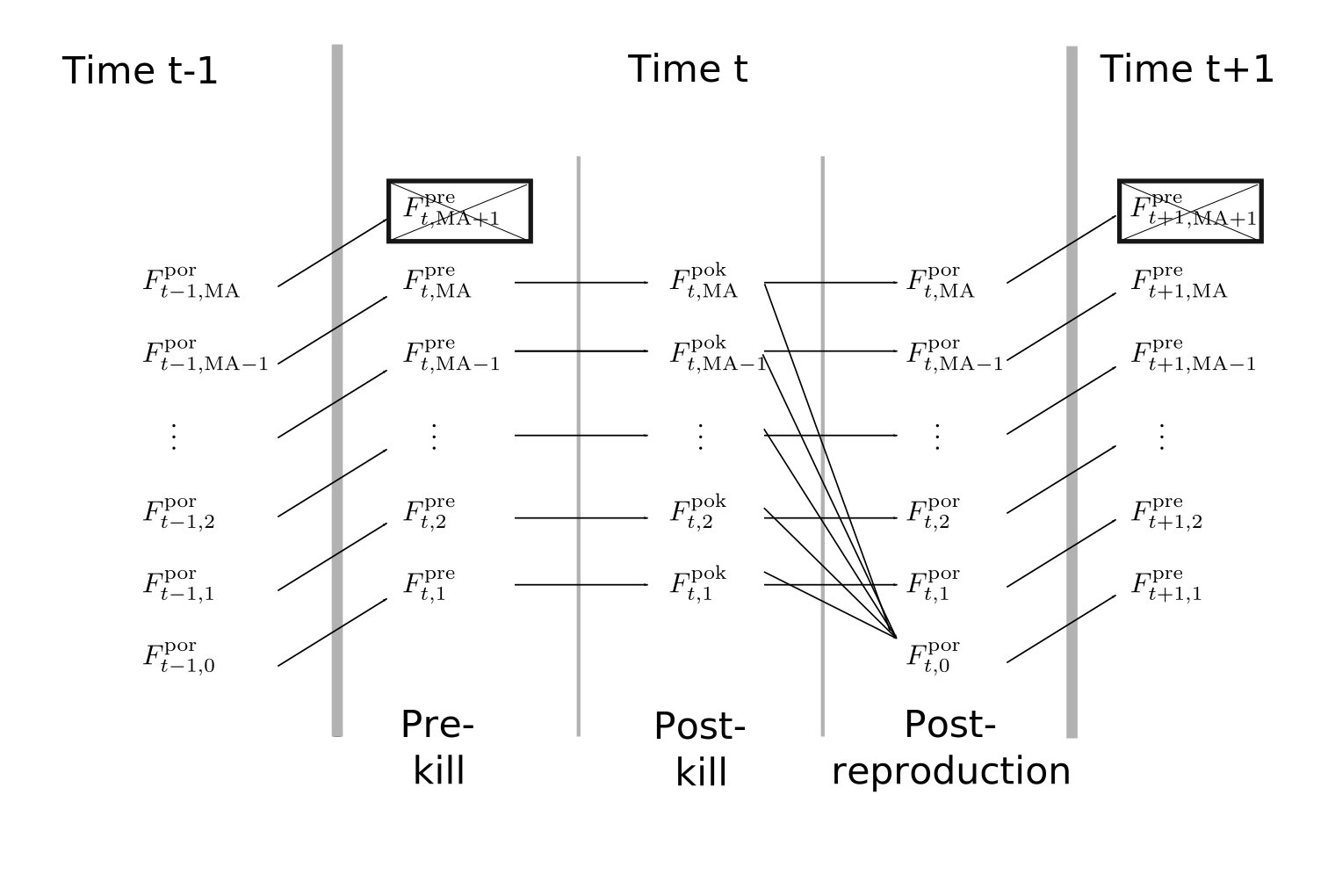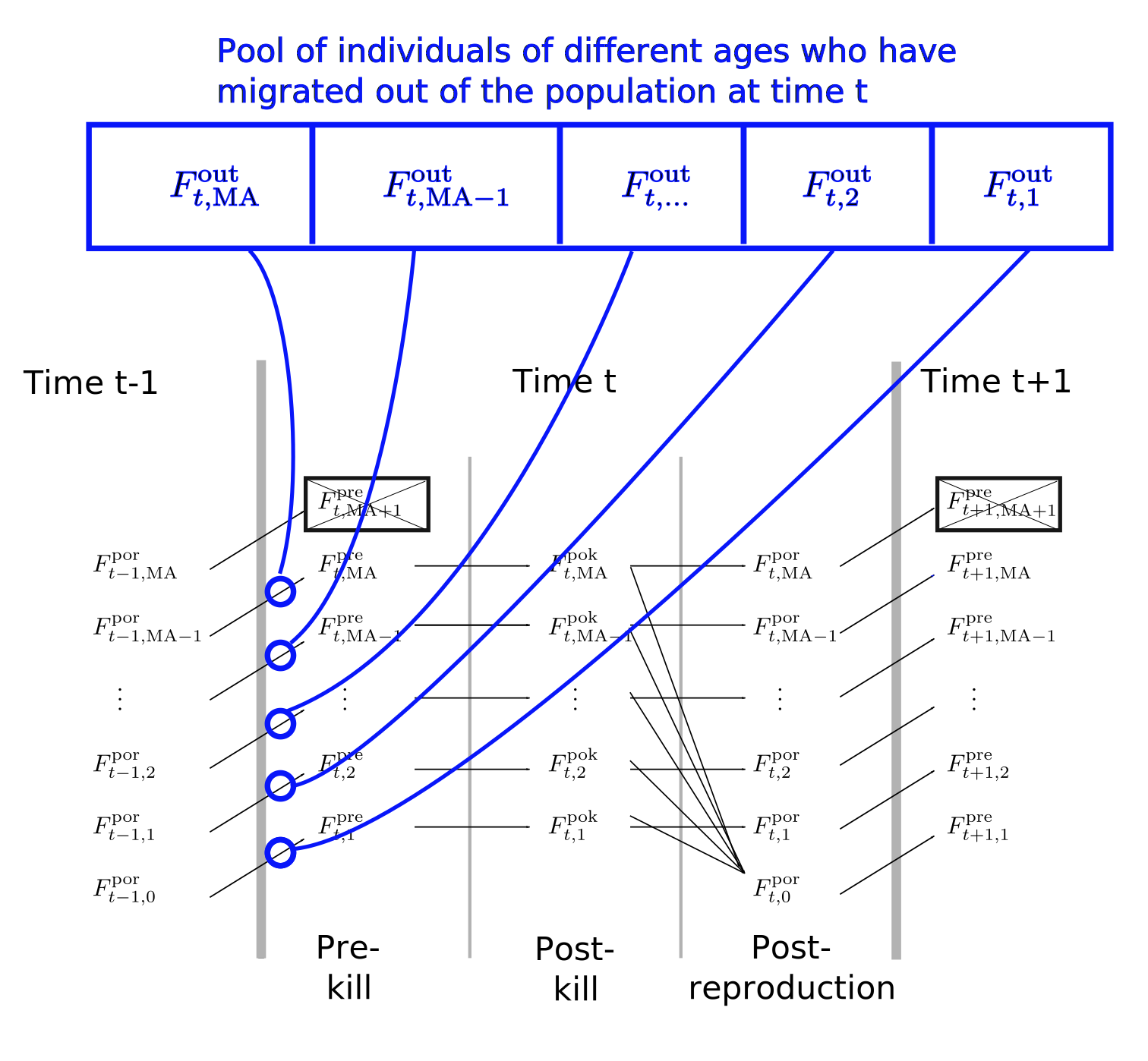#### 2021-07-13

This provides a short description of a few things that one should know about how spip works.

## Timing of events in a spip life cycle

spip is a program that records time in discrete periods that can be thought of as years. When individuals are born at time $$t$$ they are considered to be of age 0 and they have a birth year of $$t$$. The user must input to the program the maximum possible age of an individual. Here, we will refer that maximum age as $$\mathrm{MA}$$.

Within each year in spip the following events occur, in order:

1. The age of each individual is incremented at the beginning of the new year $$t$$. This means that newborns from year $$t-1$$ are turned into 1-year-olds as they enter year $$t$$, and so forth.
2. Next there is a chance to sample individuals before the death episode. Only individuals of ages 1 to $$\mathrm{MA}$$ can be sampled. These are the individuals, who, if they survive the upcoming death episode, might have the chance to contribute to the next generation.
3. Next there is an episode of death. Individuals that are $$a$$ years old in year $$t$$ survive this episode of death with a probability of $$s_a$$. Individuals that are $$\mathrm{MA} + 1$$ are all killed with probability of 1.
4. Then there is a chance to sample individuals after the episode of death. Only individuals of ages 1 to $$\mathrm{MA}$$ can be sampled. This is a sample from the individuals that are around and alive to contribute to the next generation.
5. Next there is an episode of reproduction which produces 0-year-olds born at time $$t$$.
• During the reproduction episode there is an opportunity to draw a sample from the individuals that were chosen to be amongst the reproducing adults. (This is particularly useful for critters like salmon that can be sampled explicitly when they are migrating in fresh water to reproduce).
• After the sampling period during reproduction, there is also the chance for individuals to die after reproduction according to the probability set by --fem-postrep-die and --male-postrep-die. With this value set to 1, for example, semelparity can be enforced.
6. After reproduction there are individuals of ages 0 to $$\mathrm{MA}$$ that sit around and don’t do much of anything. Eventually the year gets advanced to the following one ($$t+1$$), and the ages of individuals get incremented, so that at the beginning of year $$t+1$$ individuals are of ages 1 to $$\mathrm{MA}+1$$.

It is worth noting that even though there are some $$\mathrm{MA}+1$$-year-olds around at the beginning of each time period, they all die during the episode of death, and, because they cannot be sampled during the sampling episode before death, it is like they do not exist.

The year in spip can thus be divided into three different periods between the demographic events/episodes:

1. The period before the episode of death. This is known as the prekill period of the year, and, in the following, we will use a superscript $$\mbox{}^\mathrm{pre}$$ to denote census sizes during that period.
2. The period after the episode of death, but before reproduction. This is known as the postkill period, and will be denoted with a superscript $$\mbox{}^\mathrm{pok}$$.
3. The period after reproduction, but before the year gets incremented. This is the post-reproduction period and will be denoted with a superscript $$\mbox{}^\mathrm{por}$$.

We will use $$F_{t,a}^\mathrm{pre}$$, $$F_{t,a}^\mathrm{pok}$$, and $$F_{t,a}^\mathrm{por}$$ to denote the number of $$a$$-year-old females during the prekill, postkill, and post-reproduction periods, respectively, of time $$t$$. The diagram below, showing these numbers in relation to one another, along with notations of their expected values should help users to understand the spip annual cycle. The numbers of males change across time periods in a similar fashion.Annotated in the above are the three distinct periods in spip’s annual cycle: the prekill, postkill and post-reproductive periods. When the output of spip is slurped up by CKMRpop, these numbers become the respective tibbles (elements of the output list of slurp_spip()) of: census_prekill, census_postkill, and census_postrepro, respectively.

The expected numbers of individuals after each transition is as follows:

• $$F_{t,a}^\mathrm{pre} \equiv F_{t-1,a-1}^\mathrm{por}~~,~~a = 1,\ldots,\mathrm{MA}$$
• $$E[F_{t,a}^\mathrm{pok}] = s_a^F F_{t,a}^\mathrm{pre}~~,~~a = 1,\ldots,\mathrm{MA}$$, where $$s_a^F$$ is the probability that an $$a-1$$ year old female survives to be an $$a$$ year old female, as given with the --fem-surv-probs option.
• $$E[F_{t,a}^\mathrm{por}] = (1 - r_a^Fd_a^F) F_{t,a}^\mathrm{pok}~~,~~a = 1,\ldots,\mathrm{MA}$$, where $$r_a^F$$ is the probability that an $$a$$-year-old female reproduces (set with the --fem-prob-repro option) and $$d_a^F$$ is the probability that an $$a$$-year-old female will die after engaging in reproduction (even if no offspring were actually produced!), as given in the --fem-postrep-die option. This is an additional source of death that is useful for modeling anadromous species whose reproductive journey incurs a substantial cost.

## Sampling episodes in a spip annual cycle

The two main sampling schemes available in spip are keyed to these different time periods within the spip annual cycle as shown by the following figure:Thus, --gtyp-ppn-fem-pre and --gtyp-ppn-male-pre involve sampling from the simulated population at a different point in the year than do the --gtyp-ppn-fem-post and --gtyp-ppn-male-post options.
It is also possible to only sample those individuals that are trying to reproduce in a certain year using a third sampling scheme requested with the --gtyp-ppn-fem-dur and --gtyp-ppn-male-dur options to spip. The probability that an individual would try to reproduce in a given year is age specific and is set using the --fem-prob-repro and --male-prob-repro options.

It is worth noting that the pre, post and dur, sampling options all occur relatively independently (so long as sampling is not lethal—see the somewhat experimental --lethal-sampling option). spip reports the different years when an individual is sampled during the pre, post, and dur periods in the year. CKMRpop preserves those times in separate lists when it slurps up the spip output. For example slurped\$samples has the list columns: samp_years_list_pre, samp_years_list_post, and samp_years_list_dur. For all downstream analyses, CKMRpop uses the list column samp_years_list, which, by default is the same as the samp_years_list_post. This means, at the present time, you should use the options to sample individuals after the episode of death using the the --gtyp-ppn-fem-post and --gtyp-ppn-male-post options.

Note that, in most cases when exploring CKMR, the user will want to use the --gtyp-ppn-fem-post and --gtyp-ppn-male-post options, anyway, because those are samples from the adult population that are available for reproduction.

If it is desired to sample all newborns at time $$t$$, then currently the way to do that is to sample 1-year-olds at time $$t+1$$ using the --gtyp-ppn-fem-pre and --gtyp-ppn-male-pre options. However, it would take some extra finagling to get those sampling years into the samp_years_list column referenced above for the downstream analyses.

TODO ITEM: combine sampling at all times into the single samp_years_list column, perhaps, or make it easier for users to decide how to combine those different sampling episodes. For now, though, users should stick to using the --gtyp-ppn-fem-post and --gtyp-ppn-male-post options.

## How inter-population migration occurs in spip

We can use the same diagrams developed above to describe how migration is implemented in spip. Migration in spip is a “two-stage” phenomenon: in the first stage, individuals leave a population with sex-, year- and age-specific out-migration rates specified with the population’s options --fem-prob-mig-out and --male-prob-mig-out. They leave each population before the prekill census occurs and also before the prekill sampling occurs. Diagrammatically, it looks like this:Schematic describing the first stage of migration: migration out of a population. Each blue line shows individuals leaving the population and entering a pool of migrants.

The expected numbers of individuals in the pool of migrants who have left the population is given by the time- and age-specific rates set by the user. We will denote the outmigration rate for age $$a$$ individuals at time $$t$$ from a given population by $$m^\mathrm{out}_{t,a}$$. It follows then that, for this given population: $E[F^\mathrm{out}_{t,a}] = m^\mathrm{out}_{t,a}F_{t-1,a-1},~a=1,\ldots, \mathrm{MA}.$

In the following, we will want to refer to these outmigration rates for each population, so we may also adorn the notation, thus: $E[F^{\mathrm{out},i}_{t,a}] = m^{\mathrm{out},i}_{t,a}F^i_{t-1,a-1},~a=1,\ldots, \mathrm{MA}.$

to refer to rates and sizes specifically for population $$i$$.

After the outmigration stage, each population has a pool of migrants that are waiting to migrate into other populations. The rates by which this happens are specified with the --fem-prob-mig-in and --male-prob-mig-in options. These options set in-migration rates for different years and for different ages, effectively setting the fraction of the total number of out-migrated individuals from population $$i$$ of age $$a$$ at time $$t$$, $$F^{\mathrm{out},i}_{t,a}$$, that will migrate into the other populations. Thus, there is one number to set for each population. For example, if there are $$K$$ populations, we would have: $m^{\mathrm{in},i}_{t,a} = [m^{\mathrm{in},i}_{t,a,1},\ldots,m^{\mathrm{in},i}_{t,a,K}]~~,~~ \sum_{j=1}^K m^{\mathrm{in},i}_{t,a,j} = 1.$ The probability of migrating back to the population from whence one came is always 0. So, even if the user sets that to some non-zero value, it will be forced to zero and the values of the remaining in-migration rates will be re-scaled so as to sum to 1.

Given this set up, the expected number of individuals from the outmigrant pool from population $$i$$ that will arrive in population $$j$$, of age $$a$$ at time $$t$$ is $E[F^{\mathrm{in},i}_{t,a,j}] = m^{\mathrm{in},i}_{t,a,j} F^{\mathrm{out},i}_{t,a}$ And, so we can also write that entirely in terms of current population sizes and migration rates: $E[F^{\mathrm{in},i}_{t,a,j}] = m^{\mathrm{in},i}_{t,a,j} m^{\mathrm{out},i}_{t,a}F^i_{t-1,a-1}$

So, this whole system of specifying migrants is a little more complex than a system whereby the user specifies the fraction of individuals in population $$j$$ that originated from population $$i$$. But, it does provide a lot more control by the user, as well as realism, in that the number of migrants into a population depends on the size of the donor population.